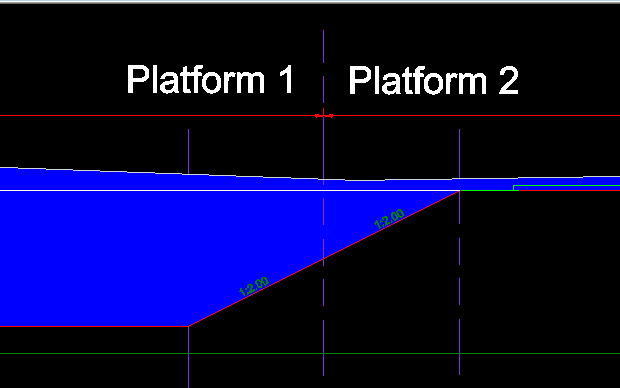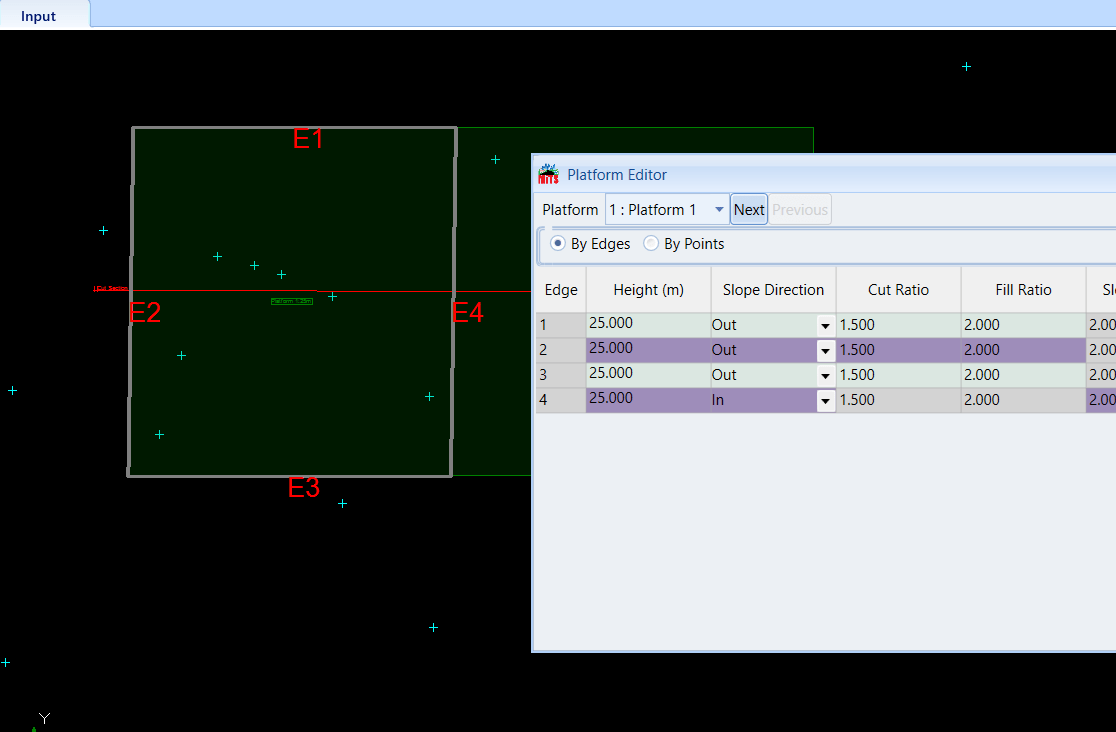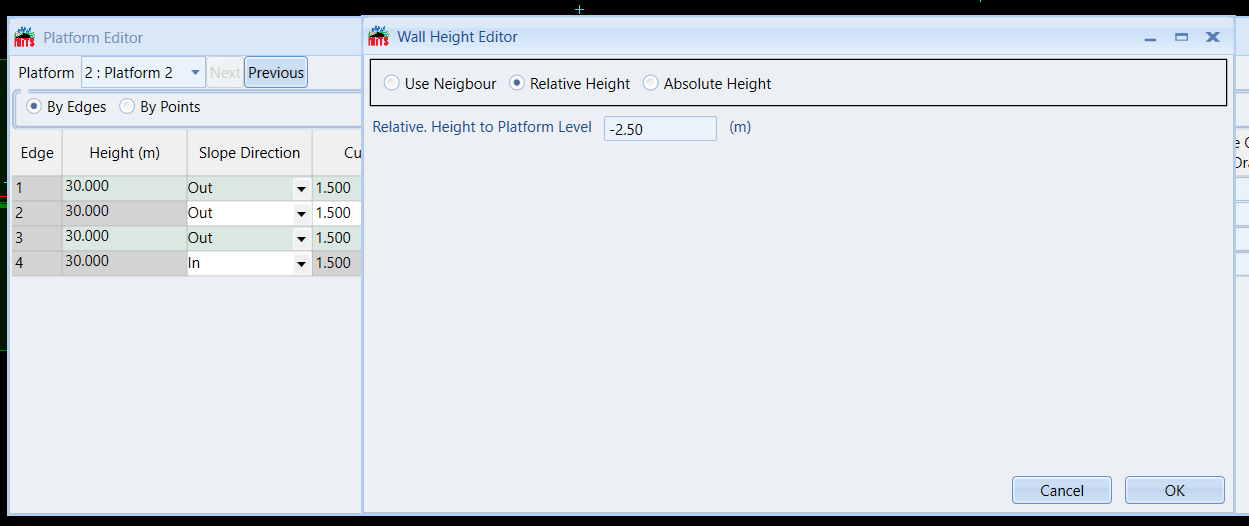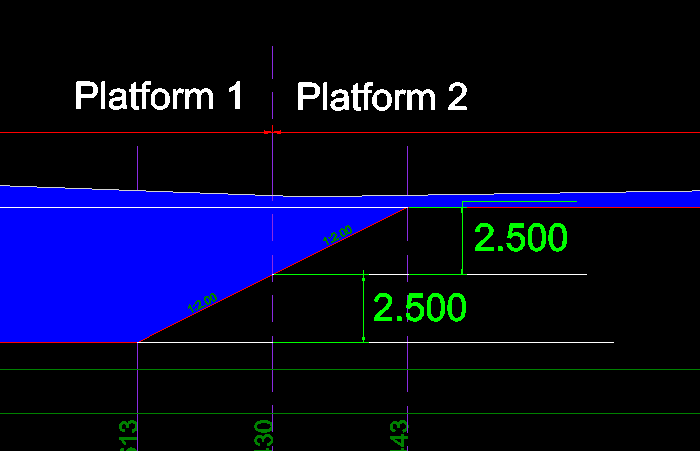603 - 5885 1250 (4 lines) info@mes100.com
Select Page

# How To Model Sharing Slope Between Conjoined Platforms

Project file to refer

Sharing centralized slope can be achieved as diagram below. The situation is when there are two connected platforms and the slopes between them are continuously connected.Platform 1 and Platform 2 are joined and the slope generated is centralized between those two slopes

In order to centralize the slope between two platforms, both of the edges have to be slope inwards. The slopes are required to be assigned as “Slope In”. Double click on the platform to come out with the Platform Editor.Edge 4 of Platform 1 is the sharing edge between the platforms, therefore the edge 4 is assigned as “Slope In”Edge 4 of Platform 2 is the sharing edge between the platforms, therefore the edge 4 is assigned as “Slope In”

There are two ways to create the sharing slope.

# Relative Height#

Relative height is the height where the slope starts. For example, if the elevation of the platform is 25 m and 2.5 m is the relative height, therefore the slope starts at 27.5 m.

Choose the sharing edge of Platform 1(in this example Edge 4 is the sharing edge). Click Slope Start Config. and choose Relative Height.2.5 m above the elevation of Platform 1 (25 m). Therefore, slope is generated inwards at 27.5 m

For Platform 2, slope also has to be connected at the same slope height of Platform 2 which is at 27.5 m. Therefore, the relative height is 2.5 m below 30 m, thus -2.5 m relative height.-2.5 m below the elevation of Platform 2 (30 m). Therefore, slope is generated inwards at 27.5 m.

Create a cut section across the platforms and Generate Slope.The slope is centralized between the platforms. 2.5 m above Platform 1 and 2.5 m below Platform 2.

# Absolute Height#

Absolute height the elevation where the slope starts. For example, if the elevation of the platform is 25 m, and you want the slope generation to start at 27.5 m, then you just simply put 27.5 m.